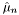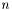#Boost C++ Libraries

...one of the most highly regarded and expertly designed C++ library projects in the world.

This is the documentation for an old version of boost. Click here for the latest Boost documentation.

## Struct template lazy_weighted_variance_impl

boost::accumulators::impl::lazy_weighted_variance_impl — Lazy calculation of variance of weighted samples.

## Synopsis

```// In header: <boost/accumulators/statistics/weighted_variance.hpp>

template<typename Sample, typename Weight, typename MeanFeature>
struct lazy_weighted_variance_impl {
// types
typedef numeric::functional::multiplies< Sample, Weight >::result_type       weighted_sample;
typedef numeric::functional::average< weighted_sample, Weight >::result_type result_type;

// construct/copy/destruct
lazy_weighted_variance_impl(dont_care);

// public member functions
template<typename Args> result_type result(Args const &) const;
};```

## Description

The default implementation of the variance of weighted samples is based on the second moment(weighted_moment<2>) and the mean(weighted_mean):

Equation 1.42.whereis the number of samples.

### `lazy_weighted_variance_impl` public construct/copy/destruct

1. `lazy_weighted_variance_impl(dont_care);`

### `lazy_weighted_variance_impl` public member functions

1. `template<typename Args> result_type result(Args const & args) const;`